Next: Some Analytically Soluble Problems Up: Brief Review of Quantum Previous: Linear Vector Spaces in

# Postulates of Quantum Mechanics

In this section, we will present six postulates of quantum mechanics. Again, we follow the presentation of McQuarrie , with the exception of postulate 6, which McQuarrie does not include. A few of the postulates have already been discussed in section 3.

Postulate 1. The state of a quantum mechanical system is completely specified by a function
that depends on the coordinates of the particle(s) and on time. This function, called the wave function or state function, has the important property that
is the probability that the particle lies in the volume element
located at
at time t.

The wavefunction must satisfy certain mathematical conditions because of this probabilistic interpretation. For the case of a single particle, the probability of finding it somewhere is 1, so that we have the normalization condition
(110)

It is customary to also normalize many-particle wavefunctions to 1.2 The wavefunction must also be single-valued, continuous, and finite.

Postulate 2. In any measurement of the observable associated with operator
, the only values that will ever be observed are the eigenvalues a, which satisfy the eigenvalue equation(111)

This postulate captures the central point of quantum mechanics--the values of dynamical variables can be quantized (although it is still possible to have a continuum of eigenvalues in the case of unbound states). If the system is in an eigenstate ofwith eigenvalue a, then any measurement of the quantity A will yield a.

Although measurements must always yield an eigenvalue, the state does not have to be an eigenstate of. An arbitrary state can be expanded in the complete set of eigenvectors of(as
(112)

where n may go to infinity. In this case we only know that the measurement of A will yield one of the values ai, but we don't know which one. However, we do know the probability that eigenvalue ai will occur--it is the absolute value squared of the coefficient, |ci|2 (cf. section 3.1.4). This leads to our third postulate.

Postulate 3. If a system is in a state described by a normalized wave function
, then the average value of the observable corresponding tois given by
(113)

Postulate 4. To every observable in classical mechanics there corresponds a linear, Hermitian operator in quantum mechanics.
This postulate comes about because of the considerations raised in section 3.1.5: if we require that the expectation value of an operatoris real, thenmust be a Hermitian operator. Some common operators occuring in quantum mechanics are collected in Table 1.

 Observable Observable Operator Operator Name Symbol Symbol Operation Position
Multiply byMomentum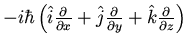Kinetic energy T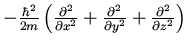Potential energy
Multiply byTotal energy E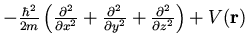Angular momentum lx
ly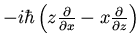lz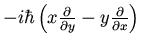Postulate 5. The wavefunction or state function of a system evolves in time according to the time-dependent Schrödinger equation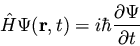(114)

The central equation of quantum mechanics must be accepted as a postulate, as discussed in section 2.2.

Postulate 6. The total wavefunction must be antisymmetric with respect to the interchange of all coordinates of one fermion with those of another. Electronic spin must be included in this set of coordinates.
The Pauli exclusion principle is a direct result of this antisymmetry principle. We will later see that Slater determinants provide a convenient means of enforcing this property on electronic wavefunctions.Next: Some Analytically Soluble Problems Up: Brief Review of Quantum Previous: Linear Vector Spaces in